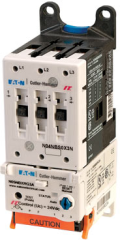Cutler-Hammer Electro-Mechanical Motor Starter. Source: Omega Engineering, Inc.DC motor starters allow a DC motor or motor-controlled device to be turned on or off.

Starting a DC motor poses unique challenges. The primary issue is that DC motors require a large, potentially damaging starting current, which must be limited by the starter itself. Whereas small motors can be started using a switch or contactor, large ones require a dedicated starter like the ones described on this page. (Find DC Motor Starters by Specification or see Directory of Suppliers.)

### Starting current

A DC motor's starting current can be determined by examining the relationship between the motor's speed, counter-electromotive force (CEMF), supply voltage, armature current and armature resistance. A motor's operational voltage can be expressed using the following formula:

E = Eb + IaRa

where:

E=voltage

Eb=counter-electromotive force

Ia=armature current

Ra=armature resistance

A motor's CEMF, or Eb in the formula above, represents the voltage that pushes back against the current that induced it. CEMF is directly proportional to the motor's speed. Since speed is zero before starting, the pre-start CEMF is also zero. Assuming that Eb=0, the formula for starting current can be derived from the above equation as Ia=E/Ra.

In practical situations, the armature resistance is kept as low as possible to maintain optimum operation of the motor. Assuming that Ra=0.5 Ω and the supply voltage E is also as low as practically possible (220 V), the minimum starting current is 440 A. This current is large enough to easily damage the armature circuit and produce high starting torque capable of spinning the rotor winding from the slots.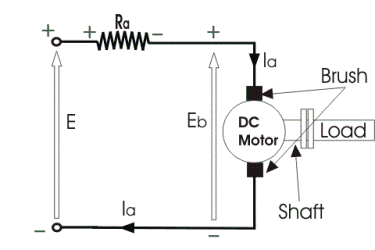The interaction of a number of variables on a DC motor.

The problem of large starting current can be solved by adding a source of external resistance that lowers the initial current. With a source of resistance, the formula for starting current is modified to: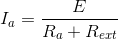where Rext=external resistance. As the motor's speed increases, CEMF develops and decreases the supply voltage, further dampening the armature current. As CEMF increases, the external resistance is slowly decreased until it reaches zero, which is the point of maximum CEMF.

Therefore, a DC starter's primary role is to safeguard the motor's circuitry by lowering the starting current through resistance. Starter designs that accomplish this are discussed below.

### Starter types

Three-point: Three-point motor starters limit starting current by providing variable resistance. These devices are so named because they connect to the motor using three terminals: the armature (A) terminal connected to the armature winding; the line (L) connected to the positive supply pole; and the field (F) terminal linked to the field winding.

As discussed above, the primary purpose of a DC motor starter is to provide resistance proportional to the amount of generated CEMF. Three-point starters accomplish this by providing variable resistance at five studs along the path of the starter handle.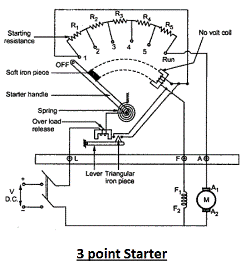A three-point starter connects to the motor using three terminals. Source: Electrical4uIn order to start the motor, the spring-loaded starter handle is moved from OFF to the first stud position. At this point, the first resistor (R1 above) provides high starting resistance, as CEMF has not yet developed. As the handle is slowly moved toward the RUN position, series resistance decreases as the motor gains speed and CEMF increases. When the handle reaches the RUN position, resistance is eliminated.

The handle remains in the RUN position against the force of the spring because the no voltage coil (NVC) shown above is magnetized when current flows through the starter. The NVC also acts as a safeguard during motor operation. If the motor's voltage supply is cut for any reason, the NVC demagnetizes and the handle spring returns the handle to the OFF position, effectively cutting the motor.

The fact that the no voltage coil is magnetized by the field current represents the drawback of three-point starters. A motor's speed is controlled by current changes effected by the field rheostat; because the NVC relies on the constant supply of field current, speed changes may result in the demagnetization of the NVC, the release of the starter handle, and the unintentional cutting of the motor. Four-point starters overcome this problem by adding an additional terminal.

Four-point: Four-point starters are identical to three-point devices.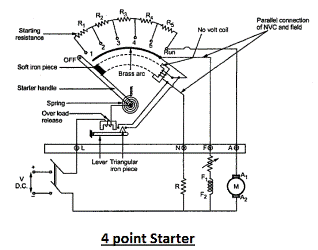A four-point starter adds an additional terminal that links the supply to the no voltage coil. Source: Electrical4uThese starters are typically employed in applications where the motor is expected to operate above normal speed. The N terminal is necessary because higher speeds reduce field currents through the F terminal so that the handle is unable to remain in the RUN position. By establishing an independent current supply to the NVC, the motor can run continuously regardless of field current and will not be unnecessarily tripped by high-speed operation or speed changes.

Series motor starters: While three- and four-point starters are typically used to start shunt and compound motors, four-point starters (with disconnected F terminals) can also be used to start series motors, provided the starting current does not exceed the starting resistor's rated current.

Dedicated series motor starters are essentially simplified versions of a three-point starter. They involve two terminals: the line (L) connecting the supply positive and the starting handle, and the field/armature connection to the motor itself. These devices are sometimes called two-point starters for this reason. Series starters are identical to three- and four-point types in operation: the handle is simply moved from OFF to RUN, at which point it remains there using the magnetized NVC.

Electronic starters: All of the motor starters above are manually controlled in that the starter handle is moved by an operator. While these starters are still in use, electronically controlled soft starters have been introduced more recently.

These devices are typically used to start shunt motors and feature a resistor series with parallel thyristors. When the motor starts, the high startup current flows through the entire resistor series so that resistance is maximized. A microcontroller then sends voltage pulses at preset intervals to fire the thyristors in sequence, progressively shunting the current around the resistors as CEMF develops. Like the manual starters above, the starter limits the potentially damaging startup current spike and starts the motor with "soft" acceleration.